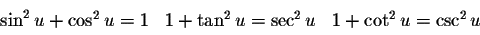# Trig Identities

## Homework Statement

Prove the Identity: tan²θ - sin²θ = tan²θsin²θ

## The Attempt at a Solution

Well I got up to (sin²θ/cos²θ) - (sin²θcos²θ/cos²θ) = sin⁴θ/cos²θ

I got the answer cause my calculator says sin²θ - sin²θcos²θ = sin⁴θ

But I don't know how sin²θ - sin²θcos²θ = sin⁴θ.

How do you subtract sin²θcos²θ with sin²θ?

Also while I'm at it, how does sin⁴θ - cos⁴θ = 1 - 2cos²θ?

Last edited:

[

## The Attempt at a Solution

Well I got up to (sin²θ/cos²θ) - (sin²θcos²θ/cos²θ) = sin⁴θ/cos²θ

I got the answer cause my calculator says sin²θ - sin²θcos²θ = sin⁴θ

But I don't know how sin²θ - sin²θcos²θ = sin⁴θ.

How do you subtract sin²θcos²θ with sin²θ?
Pull the common term out and simplify.

Also while I'm at it, how does sin⁴θ - cos⁴θ = 1 - 2cos²θ?

Realise that sin⁴θ - cos⁴θ can be written as a difference of squares, and that there is an identity which lets you write expressions of the form (a^2-b^2) in terms of the sum and difference of a and b.

Pull the common term out and simplify.

Realise that sin⁴θ - cos⁴θ can be written as a difference of squares, and that there is an identity which lets you write expressions of the form (a^2-b^2) in terms of the sum and difference of a and b.

I don't know what you mean. I'm supposed to remove the sin²θ from both sides? How do I do that? Divide both sides by sin²θ? Then wouldn't that become 1 - cos²θ which only equals to sin²θ?

Last edited:
I don't know what you mean. I'm supposed to remove the sin²θ from both sides? How do I do that? Divide both sides by sin²θ? Then wouldn't that become 1 - cos²θ which only equals to sin²θ?
sin²θ - sin²θcos²θ = sin²θ(1 - cos²θ) = sin⁴θ

symbolipoint
Homework Helper
Gold Member
Cyborg31,

You are almost done. You are just one step away. Just FACTOR what you showed on the right-hand side. Notice you have sine squared as factor!

Sorry for asking a stupid question.

But how did you get:
sin²θ - sin²θcos²θ
=sin²θ(1 - cos²θ)

Thanks.

Integral
Staff Emeritus
Gold Member
It isn't that hard.

$$tan^2 \theta - sin^2 \theta = \frac {sin^2 \theta} {cos^2 \theta} - sin^2 \theta$$

$$= sin^2 \theta ( \frac 1 {cos^2 \theta} -1)$$

Can you finish?

Last edited:
It isn't that hard.

$$tan^2 \theta - sin^2 \theta = \frac {sin^2 \theta} {cos^2 \theta} - sin^2 \theta$$

$$= sin^2 ( \frac 1 {cos^2 \theta} -1)$$

Can you finish?

Well... no, cause that's basically what I'm asking.

I know how to get that far, it becomes sin²θ - sin²θcos²θ which I don't know the steps to get it to sin⁴θ... I guess it's like I don't understand/remember how to get x^2 - (x^2*y^2) = x^4?

Do you understand that (ab - ac) = a(b - c)?

symbolipoint
Homework Helper
Gold Member
Back to your original work, remember two very fundamental identities:

Tan(b) = sin(b)/cos(b)
.....and......
cos(b)*cos(b) + sin(b)*sin(b) = 1 [please excuse lack of typesetting]

That last identity directly gives you:
sin(b)*sin(b)=1-cos(b)*cos(b) which you can use in your last step.Are you aware of these identities? And you know that (1/cos x) is the same thing as (sec x)?
..
With those identities.. you should be able to change
https://www.physicsforums.com/latex_images/12/1264215-1.png [Broken]
to
[PS Integral, you forgot the "theta" after sin^2]
[sin^2 theta][sec^2 theta -1]
Refer back to the 1st drawing I linked.. See which has the "sec".

And, if you can't rememberthe other two equations (you should at least remember the 1st), you can derive the 2nd by diving everything in the 1st by sin^2 theta, and likewise, to get the 3rd equation, divide everything in the 1st by cos^2 theta.

Last edited by a moderator:
Gib Z
Homework Helper
$\frac{1}{\cos x}$ is defined to be sec x.
$$\sec^2 \theta -1$$

That fits into the 2nd identity from the last post.

Lol, nm I figured out what you guys mean, just factored the sin²θ out of sin²θ - sin²θcos²θ, it was so simple... I need to work on factoring...

Anyways, thank you guys for the help.

I got the answer cause my calculator says sin²θ - sin²θcos²θ = sin⁴θ

Just a thought - perhaps you're rusty with factoring skills because you've been relying on your (TI-89?) way too much?

Last edited by a moderator:
Integral
Staff Emeritus
Gold Member
It isn't that hard.

$$tan^2 \theta - sin^2 \theta = \frac {sin^2 \theta} {cos^2 \theta} - sin^2 \theta$$

$$= sin^2 \theta ( \frac 1 {cos^2 \theta} -1)$$

Can you finish?

I have this quesy feeling that the OP never did understand the completion of this problem.
so:

There are several ways to finish this off. One could recognize that :
$$\frac 1 {cos^2 \theta } = sec^2 \theta$$
so we have

$$= sin^2 \theta (sec^2 \theta -1) = sin^2 \theta tan^2 \theta$$

or you could do the following:
$$= sin^2 \theta ( \frac 1 {cos^2 \theta} -1)= sin^2 \theta ( \frac 1 {cos^2 \theta} - \frac {cos^2 \theta} {cos^2 \theta}) = {sin^2 \theta} ( \frac { 1 - cos^2 \theta} {cos^2 \theta}) = {sin^2 \theta}( \frac {sin^2 \theta}{cos^2 \theta})= {sin^2 \theta}{tan^2 \theta}$$

Last edited:
Just a thought - perhaps you're rusty with factoring skills because you've been relying on your (TI-89?) way too much?

Yea... that makes sense I guess since the last time I did any real factoring was over a year ago with polynomials and the rest I just punched it in my calculator.

And I understand how to complete all the trig identities now, I just never thought about factoring them, which basically solved all the problems I was getting stuck on.